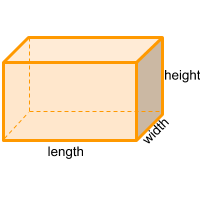Home » Polyhedra » Prisms » Rectangular Prism

# Volume of a Rectangular PrismVolume =
Height × Width × Length

## Volume calculator for a rectangular prism

Enter the Height, Width and Length of the prism to get the volume automatically.

## Description, how many faces, edges and vertices are there in a rectangular prism

The rectangular prism, also known as square prism , orthohedron, cuboid or rectangular parallelepiped, is a solid that, if it is cut in any part of its length, always maintains the shape of a rectangle.

It has 6 faces, the intersecting faces form 90 degree angles and the opposite faces are parallel, it has 12 edges and 8 vertices.

When 2 of the 3 lengths (height, width, length) are equal then we are the presence of a square prism and if the 3 lengths (height, width, length) are equal then we are in the presence of a cube or hexahedron. Still both the square prism and the cube are considered rectangular prisms.

## Examples of a rectangular prism

We can find many objects that look like an rectangular prism, examples of rectangular prism shaped objects are: a lego block, a box of milk or juice, a brick, a shoe box, a fish tank, a container, and even a building!. Can you think of any other examples? leave a comment in the comment section at the bottom of the page.

## Formula for the volume of a rectangular prism

To calculate the volume of a rectangular prism you can use the formula for all prisms, where the area of the base is multiplied by its length. In this case the base of the rectangular prism is a rectangle, therefore you need to calculate the area of the rectangle. In other words, you need to multiply the height of the rectangle, the width of the rectangle, and then the length of the prism. You can also use the online calculator to calculate the volume of the rectangular prism automatically.

Volume =
Height × Width × Length

Formula explanation:
• The formula to calculate the volume of prism is always the same:

Volume prism = Area base × Length
• In this case, the area of the base of the rectangular prism is a rectangle:

Area base = Area rectangle = Height × Width

Share